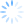Available from our resources webpage is an example spreadsheet showing how to use CDXZipStream geocoding and distance functions in an analysis of car dealership locations in the United States.  To download, just click on the link "CDXZipStream - Closing Chrysler Dealers Geocoding Analysis and Distance Matrix".  Let’s review how this works, and how you may be able to use this example in support of your own organization.

In this case there are over 700 dealerships, with locations throughout the United States, which are being considered for closing.  As part of this consideration it is necessary to calculate their relative proximity to each other.  Although it would be possible to calculate the driving distance between each location using the CDXZipStream function CDXRouteMP, a simpler and faster calculation is straight-line distance, based on the latitude and longitude of each dealership.  Since the additional accuracy of driving distance is not necessary for this analysis, we will be using straight-line distance here.

The first step, as shown in the worksheet “Dealers Closing” is to find the latitude and longitude of each dealer, either based on the exact address or by zip code.  The function CDXLocateMP is used to find the latitude and longitude in each case, such as in the following formula in columns I and J, for the first row of data:

=CDXLocateMP(1,D2,E2,F2,G2,"US")

Where

CDXLocateMP is the CDXZipStream custom function formula that finds latitude and longitude

1 indicates that latitude will be calculated and returned to the worksheet; 2 is used for longitude

D2, E2, F2, G2 are the cell addresses of the street, city, state and zip code of each dealership

“US” is the country

Since cell addresses are used in this equation, the equation can be copied down the list of dealerships to obtain latitude for all locations in all rows.

In the first row of columns K and L the formula uses zip code only:

=CDXLocateMP(1,G2)

This is also copied down the enter list of dealerships.  In cases where MapPoint cannot locate the exact dealership address, only the zip code is used where the centroid of the zip code area is the basis for the geocoding calculation.

Columns M and N uses the Excel IF and ISNUMBER functions to select the latitude and longitude of the zip code centroid when the exact address is missing:

=IF(ISNUMBER(I2),I2,K2)

In the next worksheet, called Dealer Distance Matrix, the location and latitude and longitude data (from columns M and N) of the previous worksheet are placed into a matrix to calculate the distance between all combinations of dealerships.  Straight-line distance is calculated in this matrix using the function CDXDistance2WP.  In the uppermost left hand corner of the matrix, the following formula is used:

=CDXDistance2WP(\$C5,\$D5,E\$3,E\$4)

Where

CDXDistance2WP is the CDXZipStream custom function formula that calculates distance between two sets of latitude and longitude

\$C5, \$D5, E\$3, and E\$4 are the cell addresses of the latitude and longitude pairs

The dollar sign before the column letter of row number in this equation indicates that this value does not change when the formula is copied to other areas of the worksheet.  The 789 x 789 matrix contains the distance in miles between all dealerships, across the range E5 through ADM793.  Due to the number of columns required for this example, Excel 2007 or 2010 must be used.  Excel 2003 can be used for matrices that have 256 columns or less.

The distance data ican now be effectively displayed in the worksheet “Closest Dealers” , showing dealerships according to their distance from a select dealer location.  Just use the drop down box at the top left hand side of the sheet to select a location, and all other dealerships are sorted and displayed by distance using Visual Basic for Applications code.  (Make sure that macros are enabled for this workbook, by clicking on the Macro Security icon from the Developer tab on the Excel ribbon, then select Enable All Macros.)  The VBA  code is accessible by clicking on the Visual Basic icon from the Developer tab and can be customized to suit your particular application, whether it be finding the closest competitor stores, assigning sales representatives, or targeting distribution locations by customer.